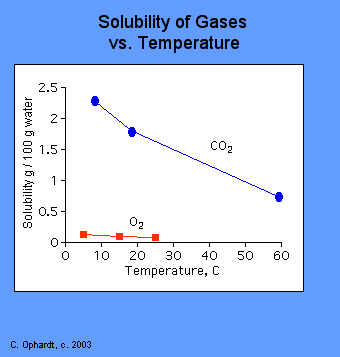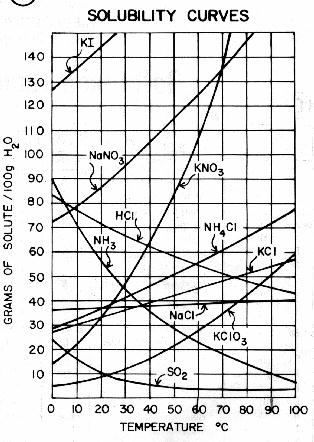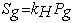Solubility

The definition of solubility is the maximum quantity of solute that can dissolve in a certain quantity of solvent or quantity of solution at a specified temperature or pressure (in the case of gaseous solutes). In CHM1045 we discussed solubility as a yes or no quality. But the reality is that almost every solute is somewhat soluble in every solvent to some measurable degree.

As stated in the definition, temperature and pressure play an important role in determining the degree to which a solute is soluble.

For Gases, solubility decreases as temperature increases (duh...you have seen water boil, right?) The physical reason for this is that when most gases dissolve in solution, the process is exothermic. This means that heat is released as the gas dissolves. This is very similar to the reason that vapor pressure increases with temperature. Increased temperature causes an increase in kinetic energy. The higher kinetic energy causes more motion in the gas molecules which break intermolecular bonds and escape from solution. Check out the graph below:As the temperature increases, the solubility of a gas decreases as shown by the downward trend in the graph.

For solid or liquid solutes:

CASE I: Decrease in solubility with temperature:

If the heat given off in the dissolving process is greater than the heat required to break apart the solid, the net dissolving reaction is exothermic (See the solution process). The addition of more heat (increases temperature) inhibits the dissolving reaction since excess heat is already being produced by the reaction. This situation is not very common where an increase in temperature produces a decrease in solubility. But is the case for sodium sulfate and calcium hydroxide.CASE II: Increase in solubility with temperature:

If the heat given off in the dissolving reaction is less than the heat required to break apart the solid, the net dissolving reaction is endothermic. The addition of more heat facilitates the dissolving reaction by providing energy to break bonds in the solid. This is the most common situation where an increase in temperature produces an increase in solubility for solids.

The use of first-aid instant cold packs is an application of this solubility principle. A salt such as ammonium nitrate is dissolved in water after a sharp blow breaks the containers for each. The dissolving reaction is endothermic - requires heat. Therefore the heat is drawn from the surroundings, the pack feels cold.

The effect of temperature on solubility can be explained on the basis of Le Chatelier's Principle. Le Chatelier's Principle states that if a stress (for example, heat, pressure, concentration of one reactant) is applied to an equilibrium, the system will adjust, if possible, to minimize the effect of the stress.  This principle is of value in predicting how much a system will respond to a change in external conditions.  Consider the case where the solubility process is endothermic (heat added). An increase in temperature puts a stress on the equilibrium condition and causes it to shift to the right.  The stress is relieved because the dissolving process consumes some of the heat. Therefore,  the  solubility  (concentration)  increases  with  an  increase  in  temperature.    If  the process is exothermic (heat given off). A temperature rise will decrease the solubility by shifting the equilibrium to the left.

Now let's look at pressure:

Solids and liquids show almost no change in solubility with changes in pressure. But gases are very dependent on the pressure of the system. Gases dissolve in liquids to form solutions. This dissolution is an equilibrium process for which an equilibrium constant can be written. For example, the equilibrium between oxygen gas and dissolved oxygen in water is O2(aq) <=> O2(g). The equilibrium constant for this equilibrium is K = p(O2)/c(O2). The form of the equilibrium constant shows that the concentration of a solute gas in a solution is directly proportional to the partial pressure of that gas above the solution. This statement, known as Henry's law, was first proposed in 1800 by J.W. Henry as an empirical law well before the development of our modern ideas of chemical equilibrium.

Henry's Law:Sg stands for the gas solubility, kH is the Henry's Law constant and Pg is the partial pressure of the gaseous solute.

#### Table: Molar Henry's Law Constants for Aqueous Solutions at 25oC

 Gas Constant Constant (Pa/(mol/dm3)) (atm/(mol/dm3)) He 282.7e6 2865 O2 74.68e6 756.7 N2 155.0e6 1600 H2 121.2e6 1228 CO2 2.937e6 29.76 NH3 5.69e6 56.9

The inverse of the Henry's law constant, multiplied by the partial pressure of the gas above the solution, is the molar solubility of the gas. Thus oxygen at one atmosphere would have a molar solubility of (1/756.7)mol/dm3 or 1.32 mmol/dm3. Values in this table are calculated from tables of molar thermodynamic properties of pure substances and aqueous solutes

Summary of Factors Affecting Solubility
Normally, solutes become more soluble in a given solvent at higher temperatures. One way to predict that trend is to use Le Chatelier's principle. Because DHsoln is positive for most solutions, the solution formation reaction is usually endothermic. Therefore, when the temperature is increased, the solubility of the solute should also increase. However, there are solutes that do not follow the normal trend of increasing solubility with increasing temperature. One class of solutes that becomes less soluble with increasing temperature is the gasses. Nearly every gas becomes less soluble with increasing temperature.
Another property of gaseous solutes in summarized by Henry's law which predicts that gasses become more soluble when their pressures above a liquid solution are increased. That property of gaseous solutes can be rationalized by using Le Chatelier's principle. Imagine that you have a glass of water inside of a sealed container filler with nitrogen gas. If the size of that container were suddenly halved, the pressure of nitrogen would suddenly double. To decrease the pressure of nitrogen above the solution (as is required by Le Chatelier's principle), more nitrogen gas becomes dissolved in the glass of water.